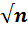Home » Subject » Management » Notes » Central limit theorem

# Central limit theorem

The Central Limit Theorem has great significance in inferential statistics. This theorem was evolved by Abraham de Moivre, a French born mathematician who used the normal distribution to approximate the distribution of the number of heads resulting from many tosses of a fair coin. The Central Limit Theorem describes the relationship between the sampling distribution of sample means and the population that the samples are taken from.

The Central Limit Theorem explains the characteristics of the "population of the means" which has been created from the means of an infinite number of random population samples of size (N), all of them drawn from a given "parent population". The Central Limit Theorem forecasts that irrespective of the distribution of the parent population (Hays, 1994):

1. The mean of the population of means is always equal to the mean of the parent population from which the population samples were drawn.
2. The standard deviation of the population of means is always equal to the standard deviation of the parent population divided by the square root of the sample size (N).
3. The distribution of means will increasingly approximate a normal distribution as the size N of samples increases.
4. When random samples of different size is taken from a population, the distribution of sample means will approach to the normal distribution. When the Sampling distribution of the mean has sample sizes of 30 or more then it is said to be normally distributed (Fischer, 2010).

The central limit theorem signifies that the sampling distribution of any statistic will be normal or nearly normal, if the sample size is large enough. Normally, a sample size is considered "large enough" in any of following conditions:

1. The population distribution is normal.
2. The population distribution is roughly symmetric, unimodal, without outliers, and the sample size is 15 or less.
3. The population distribution is moderately skewed, unimodal, without outliers, and sample size is between 16 and 40.
4. The sample size is greater than 40, without outliers.

## Central Limit Theorem Formula

In Central Limit Theorem, if random samples of n observations are drawn from any population with finite mean µ and standard deviation σ, then, when n is large, the sampling distribution of the mean X¯ is approximately normal distributed, with mean µ and standard deviation σ/√n. According to this theorem, the mean of a sampling distribution of means is an unbiased estimator of the population mean (Naval, 2010).

µx = µ

Likewise, the standard deviation of a sampling distribution of means is

 σ σx =The central limit theorem has numerous variants. In its common form, the random variables must be identically distributed. In variants, convergence of the mean to the normal distribution also happens for non-identical distributions or for non-independent observations, given that they comply with certain conditions (Hoffman, 2001). The central limit theorem concerns the sampling distribution of the sample means. Researchers may ask about the overall shape of the sampling distribution. According to the central limit theorem, this sampling distribution is approximately normal which is usually known as a bell curve. This approximation improves as researchers increase the size of the simple random samples that are used to produce the sampling distribution.

## Central Limit Theorem Main Feature

The main feature of this theorem is that a normal distribution arises regardless of the initial distribution. Even if population has a skewed distribution, which happens when researchers examine variables such as incomes or people's weights, a sampling distribution for a sample with an adequately large sample size will be normal (Fischer, 2010). It can be established that the central limit theorem described the reason of many non-normal distributions which tend towards the normal distribution as the sample size N increases. This includes uniform, triangular, inverse and even parabolic distributions.

## Central Limit Theorem Significance

The significance of the central limit theorem to statistical philosophy cannot be exaggerated. Most of hypothesis testing and sampling theory is based on this theorem. Additionally, it provides explanation for using the normal curve as a model for many naturally occurring phenomena. For example, if a trait, such as intelligence, can be thought of as a combination of relatively independent events, in this case both genetic and environmental, then it would be expected that trait would be normally distributed in a population.

To summarize, the central limit theorem states that even if a population distribution is strongly non‐normal, its sampling distribution of means will be roughly normal for large sample sizes (over 30). The central limit theorem enables to use probabilities related with the normal curve to reply questions about the means of sufficiently big samples. The central limit theorem is one of the most significant results of the theory of probability. In simple way, the theorem states that the sum of a large number of independent observations from the same distribution has, under certain general conditions, an approximate normal distribution. Furthermore, the approximation gradually improves as the number of observations increases. The theorem is considered as the probability theory.